NON-BLACKBODY RADIATION Of course, the surface is not a perfect black body; so the radiance emitted upward is oB(To), where o, a number between zero and one, is called the emittance (or emissivity) of the surface. In the infrared portion of the spectrum, for most surfaces, o is in the 0.9–1.0 range; that is, most surfaces, including land, water, and clouds, are good blackbodies. Note that perfect blackbodies reflect no radiation; all radiation incident on them is absorbed. Surfaces which are not perfect blackbodies reflect some radiation. Their reflectance is given by o = 1 – o. Since most surfaces are good emitters in the infrared, they are poor reflectors, and reflection is usually ignored. ATMOSPHERIC TRANSMITTANCE Of the radiance emitted by the surface, only a fraction makes it through the atmosphere to be measured by the satellite. This fraction is called the atmospheric transmittance (a number between zero and one). The transmittance from the surface to the satellite is o. Thus, the surface contribution to the radiance measured by the satellite is given by Lo = oB(To)o. It must be noted that o is a strong function of wavelength because atmospheric gases absorb radiation as governed by the laws of quantum mechanics and studied by the field of spectroscopy. This means that two wavelengths which differ by only a small amount can have very different transmittances. The following chart shows the transmittance of the clear (no clouds) atmosphere of Earth.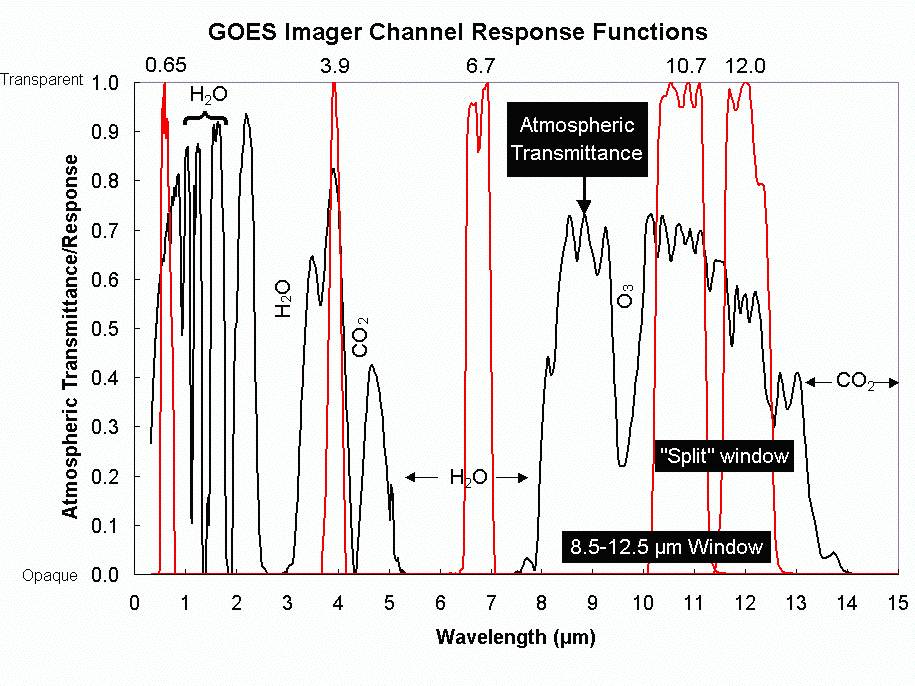GOES CHANNELS Most imager channels are located at wavelengths where the atmosphere is relatively transparent (o near 1). These transparent regions of the spectrum are called “windows” because radiation passes through them just as sunlight passes through glass windows. The 0.65 µm channel of the GOES Imager (channel 1) is in the visible window. The 10.7 and 12 µm channels of the GOES Imager (channels 4 and 5) are in the infrared window. The 3.9 µm channel (channel 2) is in a shortwave infrared window. The 6.7 µm channel (channel 3), however, is not in an atmospheric window. It does not sense the surface at all (o = 0). Rather, it senses the atmosphere. To understand what it is sensing, we must return to radiative transfer. ATMOSPHERIC RADIATION The satellite senses radiation from the surface or the atmosphere or both. Above, we considered the surface contribution. Now we must look at the atmospheric contribution. Let’s divide the atmosphere into thin layers, numbered 1 to N . Consider the radiance emitted by layer i. If the layer were a black body, it would emit radiance B(Ti), where Ti is the temperature of the layer. Since the layer is not a perfect blackbody it really emits iB(Ti), where i is the emittance (0  i  1) of the layer. A fraction of this emitted radiation i (0  i  1) reaches the satellite.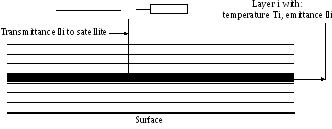THE RADIATIVE TRANSFER EQUATION Putting this all together, we can write the radiance that the satellite actually measures L = oB(To)o + iB(Ti)i. where the first term on the right is the surface contribution, and the second term on the right is the sum of the contributions for all of the atmospheric layers. This equation, usually written as an integral, is the radiative transfer equation. THE WEIGHTING FUNCTION At this point, it is customary to define Wi as Wi = ii. and to call Wi the weighting function because it “weights” (multiplies) the blackbody emission B(Ti) of layer i in the radiative transfer equation. More important than the name, however, is that the weighting function tells us how well the satellite “sees” a particular layer of the atmosphere. It is exactly what we need to know to determine where the radiation that the satellite measures comes from. If one chooses height or the logarithm of pressure (which is nearly the same as height) as the vertical coordinate, weighting functions have nearly a Gaussian shape, at least for those channels which do not sense the surface. The transmittance increases with height because at higher altitudes there are fewer absorbing molecules between the layer and the satellite. The emittance decreases with height because at higher altitudes there are fewer molecules to emit radiation (see below). The product reaches a peak somewhere, though for surface sensing channels, the peak is below the surface. The peak of the weighing function is at the level that is best sensed by the satellite. Above the peak of the weighting function, the satellite doesn't sense the atmosphere as well because there are fewer emitting molecules. Below the peak, the emitted radiation is mostly absorbed by the atmosphere above. Unfortunately, weighting functions are broad vertically, that is, they sample a thick layer of the atmosphere, which smooths out the fine structure of the vertical temperature (or moisture) profile.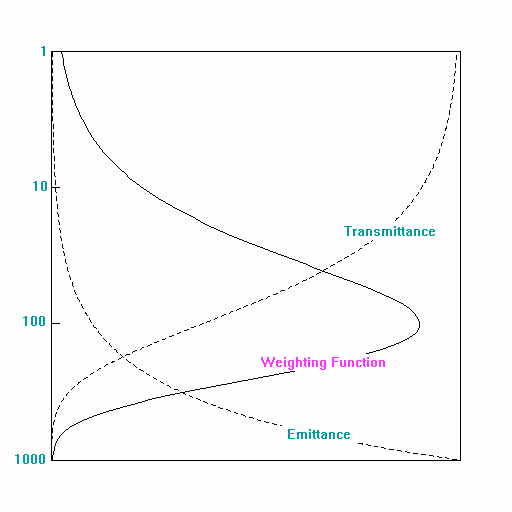The connection with absorbing gas molecules Examination of the layer emittance makes clear the connection between the gas molecules which do the absorbing and emitting. The emittance of a layer can be calculated as the product of two things: ni, the number of molecules of absorbing gas per square meter in the layer, and ai, the absorption cross sectional area of each gas molecule, i.e. i = niai Of course, ai is a strong function of wavelength and of the type of gas. In fact, if more than one absorbing gas is present in a layer, than we must add the contribution of each gas (indicated by the subscript j)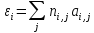What happens if we change the amount or type of gas? If we increase the amount of gas in a layer, of if we move to lower levels where the density is greater, we will increase ni and, therefore, i, as shown in the above figure. If we change the type of gas in the layer, we will radically change ai because gases absorb (or emit) at specific wavelengths determined by quantum mechanics. In any case, the layers must be chosen such that for every layer, i << 1. SOUNDING THE ATMOSPHERE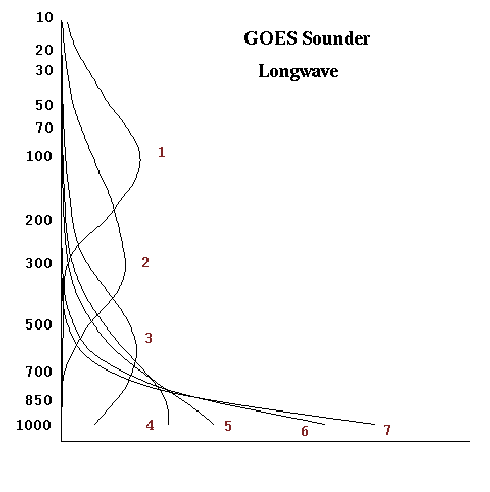By changing the wavelength, we can move the peak of the weighting function up and down in the atmosphere. By choosing a set of wavelengths which have weighting function peaks that vertically sample the atmosphere, we can get information about the vertical structure of the atmosphere. By inverting the radiative transfer equation, we can retrieve information about this structure. This is known as sounding. One difficulty is that because the weighting functions are broad, fine detail in the vertical temperature structure is lost. Note that as one changes wavelengths so that the atmosphere is more transparent, the weighting function peak moves down in the atmosphere, but the shape of the weighting function doesn't change. Eventually, the peak of the weighting function would be below the surface. This explains why surface-sensing (window) channels have an exponential shape. In truth, these weighting functions are the same as other weighting functions, but their peak is below the surface. WATER VAPOR CHANNELS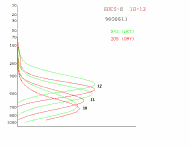If we add water vapor to a layer, it will increase the emittance of that layer, and decrease the transmittance from layers below. It will therefore move the weighting function up in the atmosphere. Conversely, if we decrease the amount of water vapor in the a layer, it will lower the emittance and lower the weighing function. This is shown in the next figure, in which the three water vapor channels for the GOES Sounder are compared in moist and dry atmospheres. The weighing functions maintain their relative shapes, but the peaks move up slightly in more moist atmospheres. WHAT ABOUT “WINDOW” CHANNELS? To conserve energy, it must be true that o + Wi = 1, that is, the satellite senses the surface or the atmosphere. For window channels (o near 1 and, therefore, Wi near zero), the radiative transfer equation can be approximated as L  oB(To)o + B(TA)(1 - o), Where TA represents temperature of the lower part of the atmosphere. This means that if one increases o, by removing water vapor, for example, then the surface is better sensed and the atmosphere is less well sensed (1 - o) decreases. This simple approximation explains why, under normal atmospheric conditions (TA < To) an infrared satellite instrument measures a window brightness temperature which is cooler than To. It also explains why when an inversion is present, the satellite can measure brightness temperatures which are warmer than the skin temperature. CONCLUSION Radiative transfer can be very complicated. If one keeps in mind a few simple concepts, however, it is not difficult to understand. Download 202.7 Kb.Share with your friends: